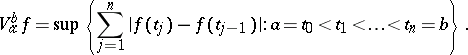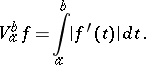# Total variation of a function

(diff) ← Older revision | Latest revision (diff) | Newer revision → (diff)

The same as the variation of a function of one variable. The total variation of a real-valued function is the sum of its positive variation (cf. Positive variation of a function) and negative variation (cf. Negative variation of a function).

Ifis a complex-valued function on, then its total variation overis the numberIfis also continuous, then this number is the same as the length of the arc in the complex plane that is parametrized by. Ifis absolutely continuous on, then